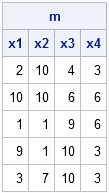I got an email asking the following question:

In the following program, I don't know how many variables are in the data set A. However, I do know that the variable names are X1–Xk for some value of k. How can I read them all into a SAS/IML matrix when I don't know the value of k?
```data _null_; call symputx("k", ceil(20*ranuni(0))); /* random number 1-20 */ run;   data a; /* create data set with k variables */ array x[&k]; do n = 1 to 5; /* 5 obs */ do i = 1 to &k; /* k vars */ x[i] = ceil(10*ranuni(1)); end; output; end; run;   proc iml; use A; read all var ??? into m; /* ??? how do I read X1-Xk ?! */ close A;```

It's a good question. In this situation, you can't specify the variables on the READ statement. Instead, restrict the variables on the USE statement by using the KEEP= option, as follows:

```proc iml; use A(keep=x:); read all var _ALL_ into m[colname=c]; close A; print m[colname=c];```The KEEP= statement drops any variables that you don't want; the _ALL_ keyword reads in all variables that remain. Consequently, the matrix m contains all variables that begin with the prefix "x" (assuming they are all numeric or all character).

ShareDistinguished Researcher in Computational Statistics

Rick Wicklin, PhD, is a distinguished researcher in computational statistics at SAS and is a principal developer of SAS/IML software. His areas of expertise include computational statistics, simulation, statistical graphics, and modern methods in statistical data analysis. Rick is author of the books Statistical Programming with SAS/IML Software and Simulating Data with SAS.

1.This will be very useful!

People send me data in all sorts of odd formats. Anything that helps read it in is very welcome!

2.Hi Rick,

Thanks for the examples. They are very helpful!
I have a quick question though. I would like to know what the
SAS error: Matrix has not been set to the value means.

The reason why I ask is because this is a vey common error that I get when I read all variables from my data sets. Thank you.

thanks

•That means that the matrix is empty. It has zero rows and zero columns. See p. 30-31 in my book, Statistical Programming with SAS/IML Software. You can download Chapter 2 for FREE from http://support.sas.com/publishing/authors/wicklin.html

3.Sachin Kumar on

Hello Rick,
Thank you for such a useful tip.
This is exactly what I was trying to find.
-Sachin